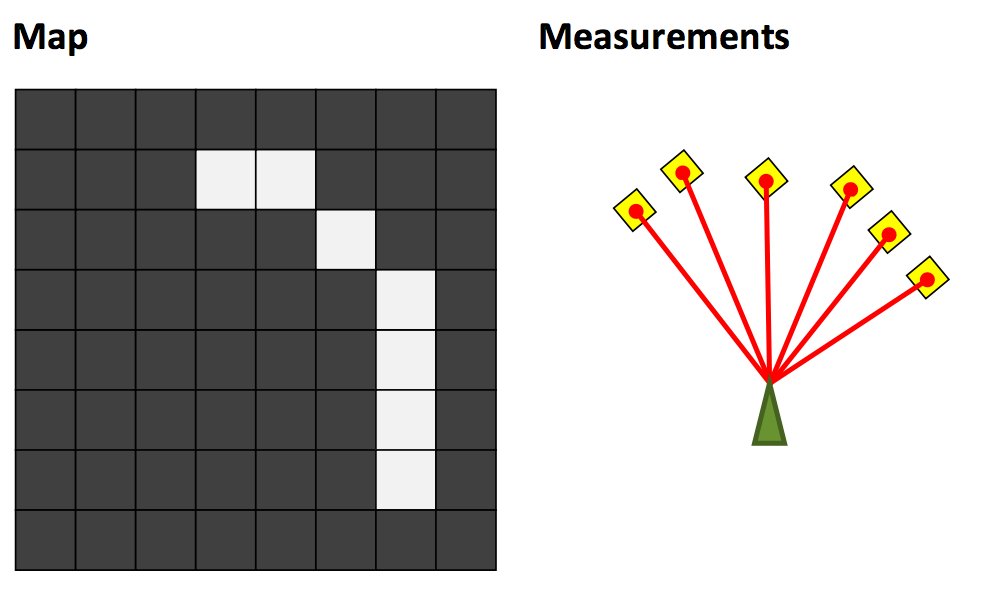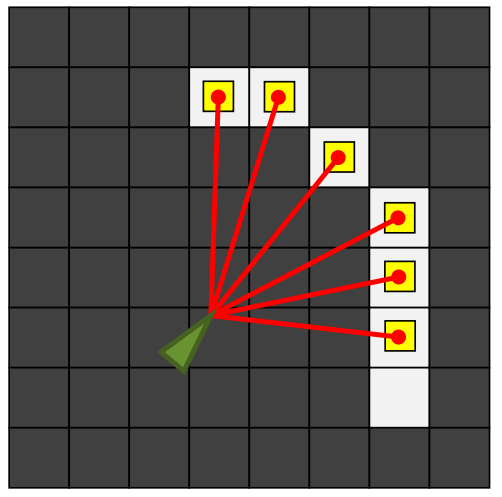## 地图定位 (Map Registration)## 粒子滤波 (Particle Filters)

1. 根据里程计的信息更新粒子的状态。
2. 由于里程计本事有不确定，更新每个粒子状态的时候可以加入里程计带入的噪音，通常也是一个高斯分布。
3. 从每个粒子更新后带噪音的分布中采样。
4. 根据距离传感器得到的信息，更新每个粒子的权重（例如由传感器得知前方近距离有障碍物，而某个粒子并没有，那么这个粒子的权重都会大幅降低）。
5. 这样得到的结果可能会有有效粒子过少的问题，需要重新采样。有效粒子的数量可以用 $$n_{effective} = \frac{(\sum_i w_i)^2}{\sum_i w_i^2}$$ 表示。
6. 重新采样的方法就是根据权重重新从粒子集中独立抽取同样数量的粒子，注意这里高权重的粒子可能会被多次抽取。重复抽取的粒子会在下一轮更新时因为里程计噪音再次分散。
7. 重复步骤，直到粒子集中在某一状态。

## 迭代最近点 (Iterative Closest Point)1. 初始化旋转 (R) 和移动 (t) 矩阵。
2. 固定 R 和 t，优化点的对应关系：$$y_i = \argmin_{y_j \in Y} \Vert x_i - y_j \Vert$$
3. 固定点的对应关系，优化 R 和 t：$$R, t = \argmin \sum_{x_i, y_i \in C} \Vert d(x_i, y_i) \Vert ^2$$
4. 重复 2 和 3，直到稳定。